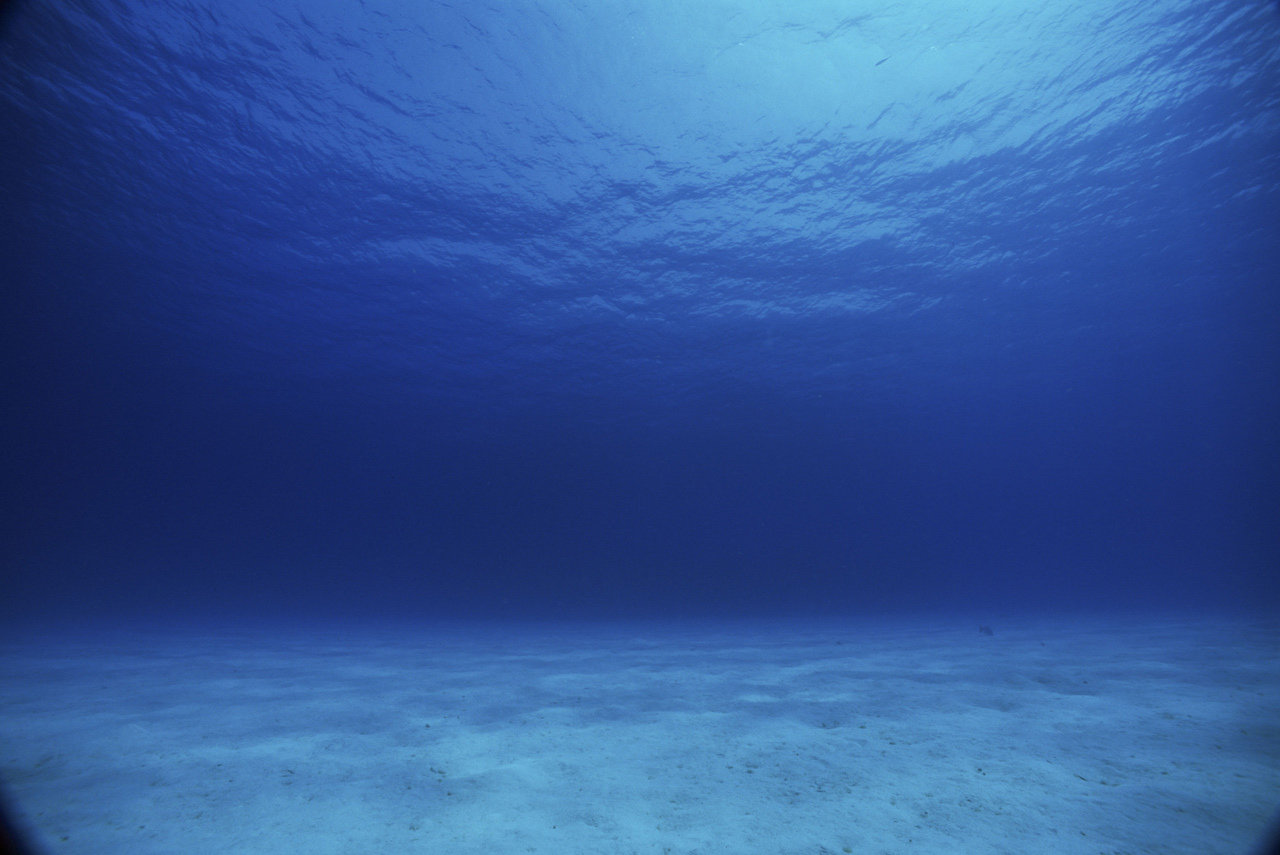## Dive-Related Info

Dive-Related Information How to calculate surface air consumption (SAC) [and RMV].

This info will be needed for OW training on upward.

Calculating Air Consumption
Air consumption is commonly expressed in two different ways: one is SAC and the other is RMV (respiratory minute volume). The two numbers express the same process in different terms, so we'll show you how to calculate both. However, in practical usage, both of the term SAC is most widely used and have come to often incorporate both meanings (SAC and RMV).

It's important to understand that an air consumption rate is a variable thing. Just like on land, you will have different values for varying levels of activity. To have the full picture, it would be a good exercise to calculate your rate at rest as well as at a relatively high state of activity (swimming) so you know the upper and lower limits of you air usage.

First, we need to record our starting and ending pressure for an entire dive or timed segment of a dive. Since we will also need to know the depth this air was consumed at, we will need to have this dive segment happen at a fixed depth, or have a way to calculate average depth for the whole dive (some dive computers will do this for you).

Example:
At the onset of a dive, we note that our submersible pressure gauge (SPG) reads 190 bar in our 10L steel tank. We descend to 5 meters and begin slowly swimming for 10 minutes. At the end of our 10 minutes, we note that our SPG reads 160 bar. With this information, we have everything we need to calculate our SAC.

During the 10 minutes, we consumed 30 bar of air (190-160)
Since SAC is expressed in minutes, we have to convert the above, so...

30 bar/10 minutes = 3 bar per minute (at 5 meters depth, or 1.5 ATA)

We then convert this to a surface value:

3 bar/min divided by 1.5 ATA = 2 bar/min SAC at sea level

This value is known as your SAC (surface air consumption) and in my estimation is not a particularly useful figure unless we always use the same size tank. However, if you did always use the same size tank (10L for example) this figure can be handy. At the surface (1 ATA) if you were breathing 2 bar/min out of your 10L tank filled to 190 bar (as in this example), you could breath for 95 minutes (190 bar ÷ 2 bar/min).

At depth, your consumption of your air supply would increase by a factor equal to the pressure. Continuing with the above example, if you were diving at 20 meters with your 10L tank, that 190 bar of air would only last 1/3 as long since our RMV would increase by 3 times due to the increased pressure and increased density of air:

2 bar/min (at 1 ATA) x 3 ATA (pressure at 20 m) = 6 bar/min at 20 m depth

So at 20 meters depth, our tank would last: 190 bar ÷ 6 bar/min = 32 min

Now, let's continue to find the RMV, which is a bit more useful since it can be universally applied to all tanks sizes. It's actually very simple. We multiply our SAC by the tank size:

2 bar/min x 10L = 20 L/min RMV (at sea level)

Metric is a very handy system for these kinds of calculations. To find out how many liters are in a tank, we simply multiply the tank volume (10L) by the current pressure (190 bar in this example), or 1900 L of air. We can now calculate what our RMV would be at different depths (20 m in this example):

20 L/min x 3 ATA (pressure at 20 m) = 60 L/min at 20 m depth

so our tank filled with 1900 L would last:

1900L ÷ 60 L/min = 32 minutes (same answer as above using SAC)

However the real beauty of RMV is we can easily use this for other tank sizes. If we were diving a 12L steel tank that was filled to 195 bar:

12 L x 195 bar = 2340 L of air in the tank

If we dive to 32 meters:

20 L/min (RMV at sea level) x 4.2 ATA (pressure at 32 m depth) = 84 L/min

2340 L (how much air we are starting with) ÷ 84 L/min = 28 minutes work of air at depth